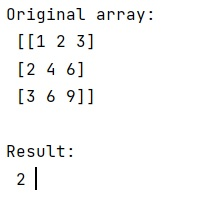# Check if numpy array is multidimensional or not

Learn, how to check if numpy array is multidimensional or not in Python?
Submitted by Pranit Sharma, on January 23, 2023

NumPy is an abbreviated form of Numerical Python. It is used for different types of scientific operations in python. Numpy is a vast library in python which is used for almost every kind of scientific or mathematical operation. It is itself an array which is a collection of various methods and functions for processing the arrays.

## Checking if numpy array is multidimensional or not

Suppose that we are given a NumPy array and we need t check whether it is multi-dimensional or not. We can also determine that if a NumPy array is multi-dimensional then what is the dimension of this NumPy array.

For this purpose, we can use the ndim property of the ndarray. It returns the number of dimensions of an array.

Let us understand with the help of an example,

## Python code to check if numpy array is multidimensional or not

```# Import numpy
import numpy as np

# Creating a numpy array
arr = np.array([[1,2,3],[2,4,6],[3,6,9]])

# Display original array
print("Original array:\n",arr,"\n")

# Check the dimensions of the array
res = arr.ndim

# Display result
print("Result:\n",res,"\n")
```

Output: